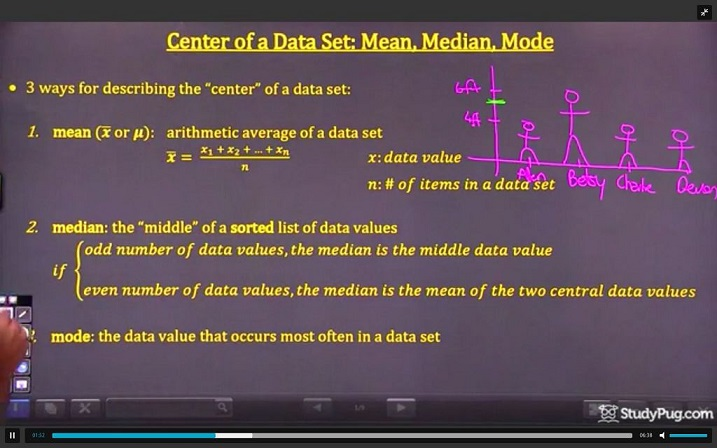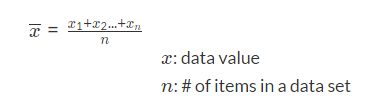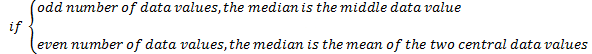# Center of a data set: mean, median, mode

## What is mean, median, and mode?

Before we start working with mean, median and mode, we should understand what they are. All three of them has to deal with averages in math. While there are lots of other averages in math, mean, median and mode are the three most common ones you'll be dealing with.

## How to find mean, median, and, mode?

To start off, mean is the average where you add together all the numbers you want to find the average of, then divide it by the count of how many numbers you added together. For the median, it's finding the number that's exactly in the middle in a list of numbers. You'll first have to put the numbers in ascending order first (with the smallest number coming first, and ordering them to the largest) before taking the middle number. Lastly, the mode is the number that shows up the most within a set of numbers. One thing to keep in mind is that a set of numbers may have no mode if there are no repeats.

Let's go through some examples to see the three averages being used.

## How to find the mean

In this question, let's pretend there are several students in a class: Alan, Betsy, Charlie, Devon.basics of mean median and mode

Their heights are as follows.

Alan = 4 feet

Betsy = 6 feet

Charlie = 4 feet

Devon = 4 feet

How would we find the mean of these four students? We can use the following formula:mean formula

Based on the formula, we'll have to add each of the data value together, then divide it by the number of items.

This will give us:

(4+6+4+4)/4= 4.5 feet

Therefore, when using the mean as the average, the average height of these students is 4.5 feet.

## How to find the median

Using the same example as above, how would we take the median of the four students? We'll first put the four students into a set of data. This gives us: {4,6,4,4}

Before finding the median, we'll have to sort the data set into ascending order. This will give us: {4,4,4,6}. If this data set had an odd number of data values, the median would have been very easy to find. It would have just been the number in the middle. However, since we have an even number of data values, we'll take the two middle numbers and divide it by two to get the average between them. This gives us: (4+4)/2

This gives us a median of 4 feet.

## How to find the mode

Let's finish learning about averages and find the mode of the students' height. The third way to figure out the center of a data set is the mode. It is the number in a data set that appears the most often. In the case of our class, we had four students: {4,6,4,4}

It's quite simple to spot the number that appears most frequently in this case. We know that the number is 4, and therefore, our mode is 4 feet.

If a data set has multiple numbers that appear the same number of times, i.e. there were also 3 students in our example's class that were 6 feet so that the data set became {4,6,4,4,6,6}, we can have two modes. You are allowed to have multiple modes in a data set, and in a case where there are two modes, your answer is bimodal.

As a parting note, while it is possible for the mean, median, and mode of a data set to have the same numbers, this isn't something that you should expect to get. In our example above, you can see that although the median and mode are both 4 feet, our mean was 4.5 feet. Depending on your data set, you can get different numbers for all three of the averages.

If you wanted to try out a game that helps teach you the mean, median, and mode, we recommend this one. If you also wanted to double check your answers, there's a online average calculator that can help.

### Center of a data set: mean, median, mode

#### Lessons

3 ways for describing the "center" of a data set:

1. mean ($\overline{x}$ or $\mu$): arithmetic average of a data set

$\overline{x}= \frac{x_1+x_2...+x_n}{n}$
$x$: data value
$n$: # of items in a data set

2. median: the "middle" of a sorted list of data values3. mode: the data value that occurs most often in a data set

$\cdot$ The median is not affected by outliers, but the mean is.

$\cdot$ weighted mean($\overline{x}_{weighted}$): arithmetic average where some data values contribute more than others

$\overline{x}_{weighted}=$ $\frac{\sum_{{i=1}}^{n}x_i \cdot w_i}{\sum_{{i=1}}^{n}w_i}$

• Introduction
a)
Overview: Mean, Median, and Mode

b)
What is weighted mean?

• 1.
Determine the mean of each set of data:
a) {8, 5, 2, 12, 3}
b) {7, 5, 3, 8, 3, 4}

• 2.
Determine the median of each set of data:
a) {8, 5, 2, 12, 3}
b) {7, 5, 3, 8, 3, 4}

• 3.
Investigating the Impact of Outliers on the Mean and Median
The heights (in cm) of students in a class are: {156, 152, 148, 159, 150}
a) Determine the mean and the median.
b) A new student with a height of 255 cm joins the class. How does the new data value (outlier)affect the mean and the median?

• 4.
Determine the mode of each set of data:
a) {8, 5, 2, 12, 5}
b) {6, 6, 6, 7, 7, 8, 8, 8, 9}
c) {1, 2, 3, 4}

• 5.
Determining the Mean of a Frequency Distribution
Determine the mean for the population of scores in the following frequency table.

 score frequency 4 6 5 3 7 8 9 2

• 6.
Determining the Weighted Mean
Sarah has earned the following averages in her statistics course: homework-98, quizzes-92, tests-84. The overall course grade is comprised of: homework (10%), quizzes (20%), unit tests (40%), and final exam (30%).
a) What is Sarah's grade going into the final exam?
b) What score must Sarah earn on the final exam in order to earn a final grade of 90% for the course?